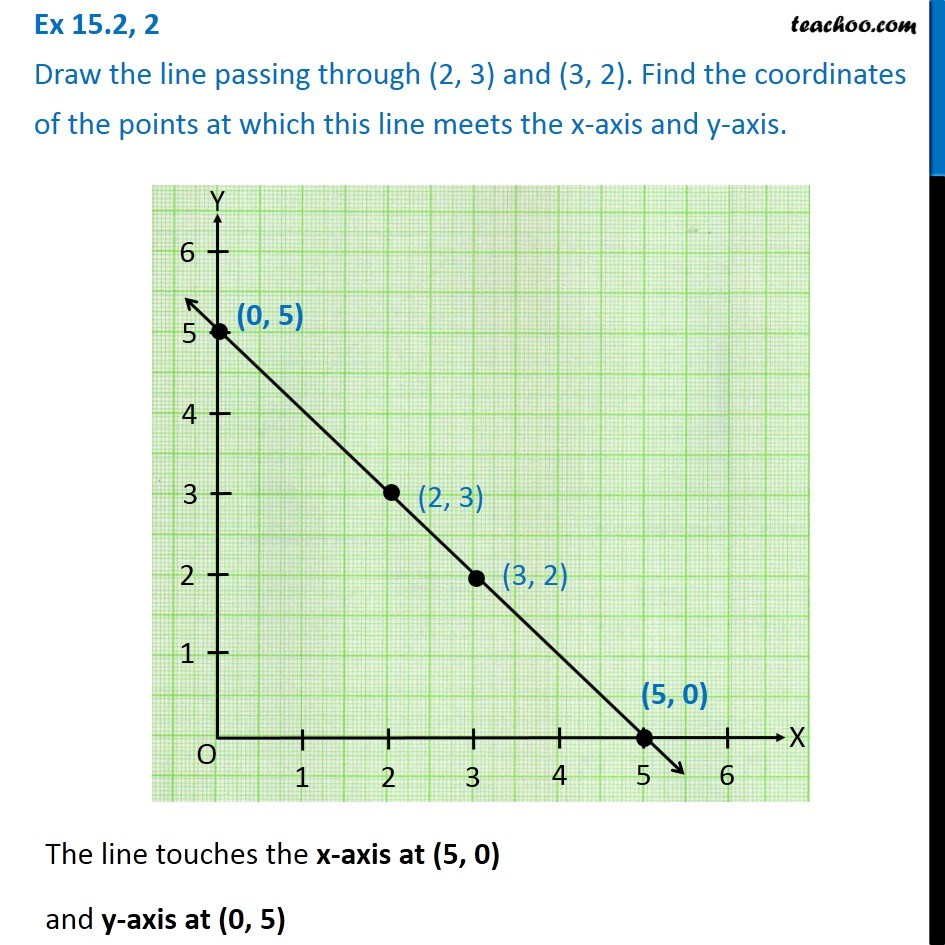1. Chapter 15 Class 8 Introduction to Graphs
2. Concept wise
3. Linear Graphs

Transcript

Ex 15.2, 2 Draw the line passing through (2, 3) and (3, 2). Find the coordinates of the points at which this line meets the x-axis and y-axis.The line touches the x-axis at (5, 0) and y-axis at (0, 5)

Linear Graphs